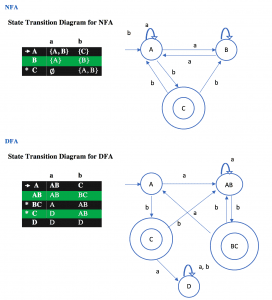## The server requested authentication method unknown to the client (SQLSTATE[HY000] )

This error occurs because of mysql  database uses caching_sha2_password so to get rid of this problem we should run.

` ALTER USER 'yourusername'@'localhost' IDENTIFIED WITH mysql_native_password BY 'youpassword'; `

It will change identification of password and solve the problem.## Solution of Realm Migration Error Code 10

When you make a change on model you have two options. You can delete app from simulator and there will not a problem after running again but if you already has shipped your app
to apple store this option is not acceptable for you. The other option is using migration.
This code is a quotation from realm.io
Suppose we have the following Person model:

```class Person: Object {
@objc dynamic var firstName = ""
@objc dynamic var lastName = ""
@objc dynamic var age = 0
}
```## Taking a character and integer from user in ASM Assembly

Question : Write an assembly program that prompts the user for a character, and then for an integer. The program then prints out “The character entered was: followed by the character, and “The integer entered was: ” followed by the integer.

```
%include 'asm_io.inc'
segment .data
msg1 db "Enter a character : ",0
msg2 db "Enter a integer : ",0
msg3 db "Entered character was : ",0
msg4 db "Entered integer was : ",0
segment .bss
integer resd 1
character resb 1
segment .text
global _asm_main
_asm_main:
enter	0,0
pusha
;---------------------------- start ponit -----------------------
mov eax,msg1 ; move msg1 to eax register
call print_string ; print to screen  msg1
mov [character],al; move the al value to character variable

mov eax,msg2 ; move msg2 to eax register
call print_string; print to screen msg2
mov [integer],al; mov al value to integer variable

mov eax,msg3; move msg3 to eax register
call print_string ; print to screen msg3
mov eax,[character]; move characker  to eax register
call print_char; print to screen charecter
call print_nl; move to point new line

mov eax,msg4; move msg4 to eax register
call print_string; print to screen msg4
mov eax,[integer]; move integer to eax register
call print_int; print to screen number
;---------------------------- start end ------------------------------
popa
mov	eax, 0
leave
ret
```## Example of Conversion of Epsilon ε-NFA to NFA – 3

Example 3:  Convert the following ε-NFA to its equivalent NFA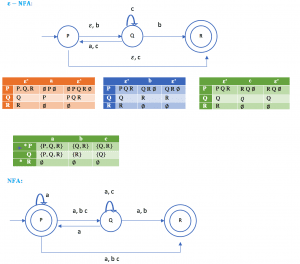## Example of Conversion of Epsilon ε-NFA to NFA – 2

Example : Convert the following ε-NFA to its equivalent NFA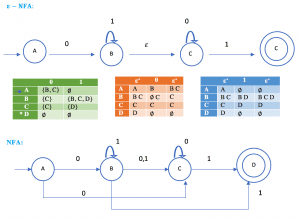## Example of Conversion of Epsilon NFA to NFA – 1

Example : Convert the following ε-NFA to its equivalent NFA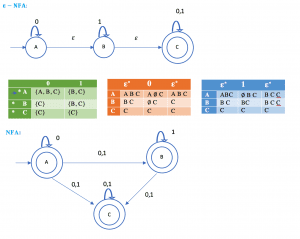## Example of Minimization of Deterministic Finite Automata (DFA)

Minimization of DFA (Table Filling Method or Myhill-Nerode Theorem)

Steps:

1. Draw a table for all pairs of states (P, Q)
2. Mark all pairs where Pϵ F and Q∉F
3. If there are any Unmarked pairs (P, Q) such that [δ(P, x),δ(Q, x)] is marked, then mark [P, Q] where ‘x’ is an input symbol. Repeat this until no more marking can be made.
4. Combine all the unmarked pairs and make them a single state in the minimized DFA.

Example: Minimize the following DFA using Table Filling Method.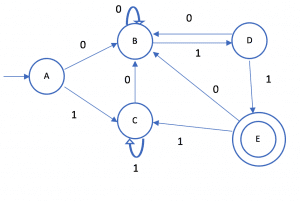## Example of Conversion of Non-Deterministic Finite Automata (NFA) to Deterministic Finite Automata (DFA) – 5

Example : Design a NFA for a language that accepts all string over {0,1}, in which the second last symbol is always 1. Then convert it to its equivalent DFA.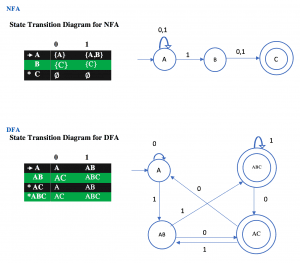## Example of Conversion of Non-Deterministic Finite Automata (NFA) to Deterministic Finite Automata (DFA) – 4

Example :Given below is the NFA for a language L= {Set of all string over (0, 1) that ends with 01 }  construct its equivalent DFA.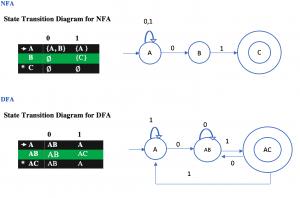## Example of Conversion of Non-Deterministic Finite Automata (NFA) to Deterministic Finite Automata (DFA) – 3

Example:  Find the equivalent DFA for the given by M= [{A, B, C}, (a, b), , A, {C}] where δ is given by: